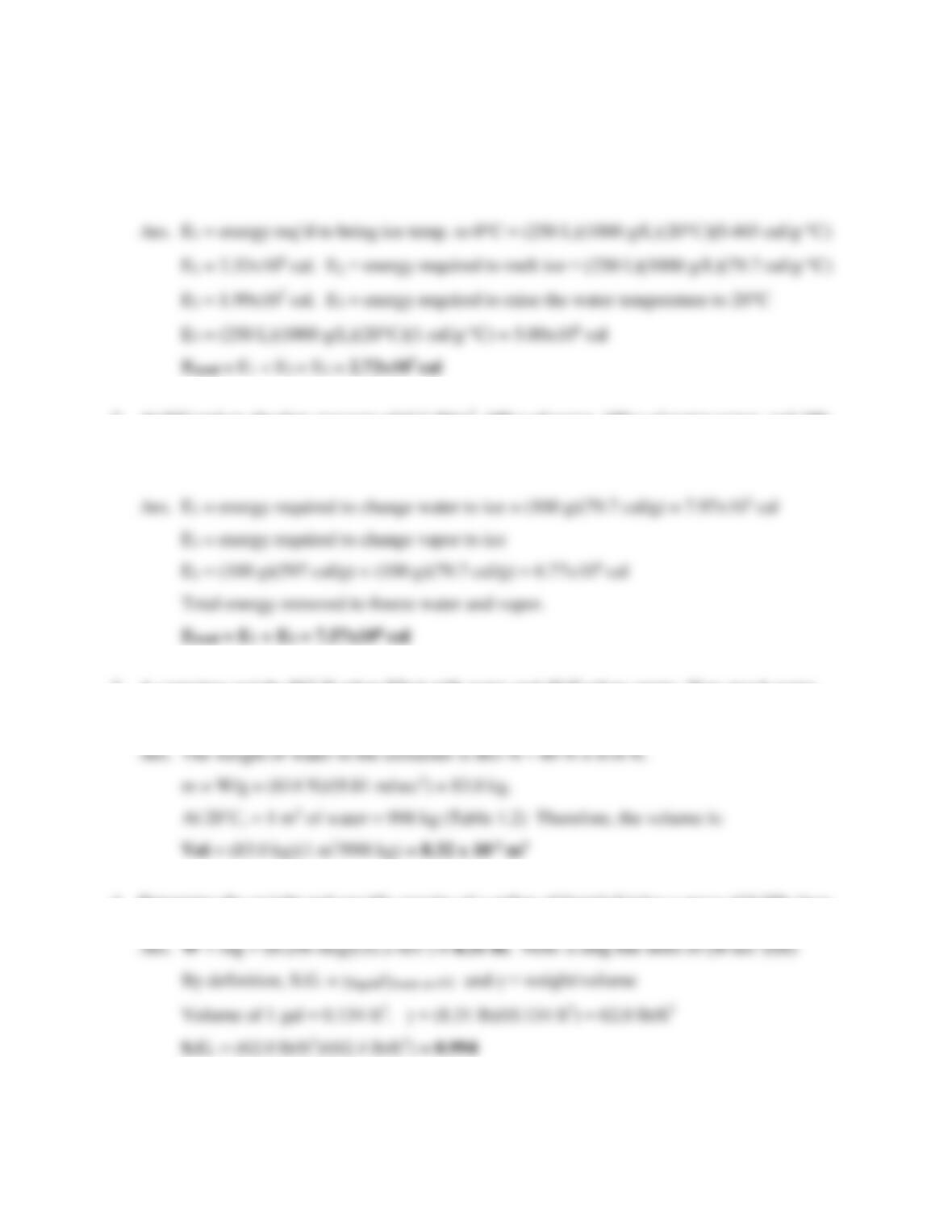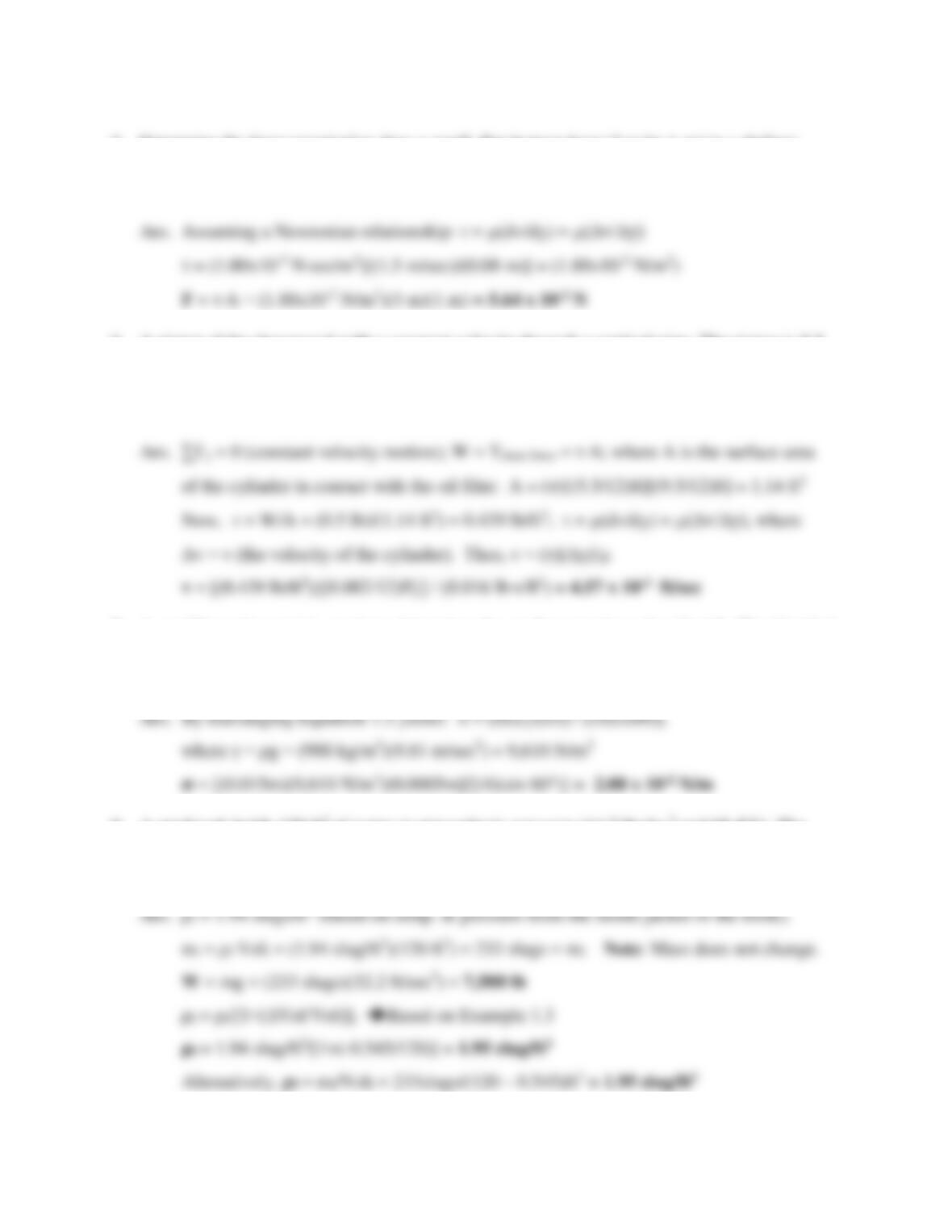# 978-0134292380 Chapter 1

Document Type
Test Prep
Book Title
Fundamentals of Hydraulic Engineering Systems 5th Edition
Authors
A. Osman H. Akan, Ned H. C. Hwang, Robert J. Houghtalen1
TEST QUESTIONS - CHAPTER #1
1. Define specific heat.
Ans. The amount of energy required to raise the temperature of a substance by 1oC.
2. Define cavitation.
Ans. Cavitation is rapid water vaporization that occurs in closed systems (e.g., pipelines or
3. The amount of energy required to change water from one phase to another is called
a) heat of fusion b) heat of vaporization c) specific heat d) latent energy
4. (T or F) To change water from one phase to another requires a change in heat or pressure.
5. Two locations in closed hydraulic systems where cavitation is likely are (pressure tanks,
pipelines, nozzles, pumps, hydraulic jacks).
6. What would be the ramification to our planet if a) water did not have a high heat capacity?
b) water did not have a high dissolving capacity? c)water did not have a unique temperature-
7. What is the difference between density and specific weight?
Ans. Density is mass per unit volume and specific weight is weight per unit volume.
8. Define specific gravity of a liquid.
Ans. Specific gravity is the ratio of the specific weight of any liquid to that of water at 4˚C.
9. (T or F) Water, like most substances, becomes denser as its temperature decreases.
Ans. False water becomes less dense at temperatures lower than 4˚C and expands even
more when it freezes.
10. (T or F) The density of ice is the same as that of liquid water at the same temperature.
11. Derive the relationship between specific weight and density using Newton’s 2nd Law (F=ma)2
12. All of the following are true except:
a) The density of water is greatest at 4˚C.
b) Specific weight can be found by multiplying density and gravitational acceleration.
c) The densities of objects on the moon are the same as they are on earth.
d) The specific weights of objects on the moon are the same as they are on earth.
13. Dividing the specific weight by the mass density of a liquid yields
a) the specific gravity of the liquid.
b) the weight of the liquid.
c) the gravitational acceleration
d) none of the above.
14. Define absolute viscosity.
Ans. Absolute viscosity is the proportionality constant relating shearing stress to the rate of
angular deformation (dθ/dt) in Newtonian fluids.
15. (T or F) Newtonian fluids were consumed in great quantities by Sir Isaac Newtonian.
16. Kinematic viscosity
a) is the proportionality constant relating shearing stress to the rate of angular deformation.
b) is expressed in units of stress times the time interval (N∙sec/m2).
c) is found by dividing the absolute viscosity by the mass density at the same temperature.
d) is not related to the density of a fluid.
17. Shear stresses in water are related to all of the following except:
a) The surface tension of the water.
b) The velocity difference between adjacent layers.
c) The distance between adjacent layers.
d) The temperature of the water.
18. Define surface tension.
Ans. Surface tension is the property of a fluid that causes it to seek a minimum possible
surface area. It occurs because fluid molecules seek to bond with other fluid particles,
but at the surface are not able to bond in all directions and therefore form stronger
19. (T or F) Determination of the capillary rise in a small tube is based on a force balance.20. Give two examples of surface tension at work (i.e., evidence of surface tension).
21. Capillary rise of water in a small tube is dependent on all of the following except:
a) the elasticity of water.
b) the nature of the solid surface.
c) the temperature of the water.
d) the diameter of the tube.
e) the angle of contact between the water and the tube.
22. Define bulk modulus of elasticity.
Ans. The bulk modulus of elasticity is the inverse of the compressibility of a fluid. Expressed
mathematically, it is the ratio of the pressure change that is exerted on a liquid to the
ratio of the change in volume over the original volume.
23. (T or F) Water is less compressible than steel, hence it is commonly assumed to be
incompressible.
24. The bulk modulus of elasticity is not dependent upon:
a) initial pressure b) initial volume c) pressure change d) volume change
Ans. a4
Problems
1. How much energy must be added to ice at -20˚C to produce 250 liters of water at +20˚C?
2. At 0˚C and an absolute pressure of 611 N/m2, 100 g of water, 100 g of water vapor, and 100
g of ice are in equilibrium in a sealed thermal container. Determine how much energy should
be removed to freeze all the water and vapor.
3. A container weighs 863 N when filled with water and 49 N when empty. How much water
(at 20˚C) does the container hold in cubic meters?
4. Determine the weight and specific gravity of a gallon of liquid if it has a mass of 0.258 slugs.5
5. Determine the force required to drag a small, flat-bottom boat (3 m by 1 m) in a shallow
canal (8 cm deep) in order to maintain a velocity of 1.5 m/sec. Assume the canal water
(20˚C) is not moving and is behaving in a Newtonian fashion.
6. A piston slides downward with a constant velocity through a vertical pipe. The piston is 5.5
inches in diameter and 9.50 inches in length. An oil film between the piston and pipe wall
resists the downward motion. If the film thickness is 0.002 in. and the cylinder weighs 0.5
pounds, estimate the velocity. (Assume the oil viscosity is 0.016 lb∙sec/ft2.)
7. A capillary rise test is used to determine the surface tension of a liquid. The liquid is
observed to rise to a height of 1.5 cm in a 0.5-mm-diameter glass tube with a contacting
angle of 60˚. Determine the surface tension of the liquid in N/m if its density is 980 kg/m3.
8. A steel tank holds 120 ft3 of water at atmospheric pressure (14.7 lbs/in.2 and 68.4˚F). The
water is subjected to a 100-fold increase in pressure. Determine the initial weight and final
density of the water if the volume decreases by 0.545 ft3.

## Trusted by Thousands ofStudents

Here are what students say about us.